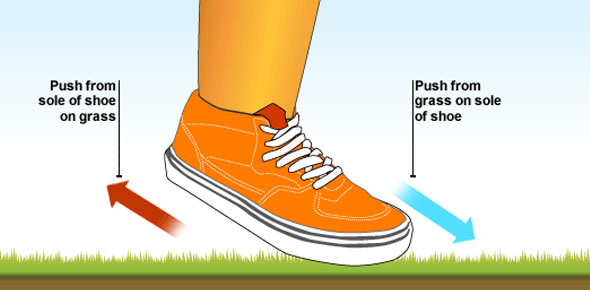10 QuestionsSettingsWaves are all around. Whether we see it or not, we experience waves every day in the form of Sound waves, visible light waves, radio waves, microwaves, water waves, sine waves, cosine waves, earthquake waves, etc. This quiz has been developed for physics students and learners to test their knowledge about wave and waves motion. So, let's try out the quiz. All the best!

Related Topics
• 1.
A wave has a speed of 10 m/s and a frequency of 100 Hz. What is its wavelength?
• A.

0.1 meters

• B.

1 meter

• C.

10 metrs

• D.

100 meters

• 2.
A wave with a frequency of 1000 Hz has a period of
• A.

0.001 seconds

• B.

0.0001 seconds

• C.

0.01 seconds

• D.

0.1 seconds

• 3.
In a longitudinal wave, the motion of the particles is _____ the wave's direction of propagation.
• A.

Parallel to

• B.

Perpendicular to

• C.

Along

• D.

Opposite from

• 4.
A sound wave is an example of a _____ wave.
• A.

Rayleigh

• B.

Interrupted

• C.

Longitudinal

• D.

Transverse

• 5.
Time taken to complete a wave is termed as
• A.

Span

• B.

Period

• C.

Life

• D.

Duration

• 6.
Any two shortest points in a wave that is in phase are termed as
• A.

Wave distance

• B.

Wavelength

• C.

Phase length

• D.

Amplitude

• 7.
Motion that is repeated at regular intervals is termed as
• A.

Vibration

• B.

Oscillation

• C.

Ventilation

• D.

Periodic motion

• 8.
Wave motion in a medium transfers
• A.

Mass, only

• B.

Energy, only

• C.

Neither mass nor energy

• D.

Both mass and energy

• 9.
As the frequency of a wave increases, the period of that wave
• A.

increases

• B.

Remains the same

• C.

Decreases

• D.

None

• 10.
An opera singer's voice is able to break a thin crystal glass if the singer's voice and the glass have the same natural
• A.

Speed

• B.

Amplitude

• C.

Wavelength

• D.

frequency